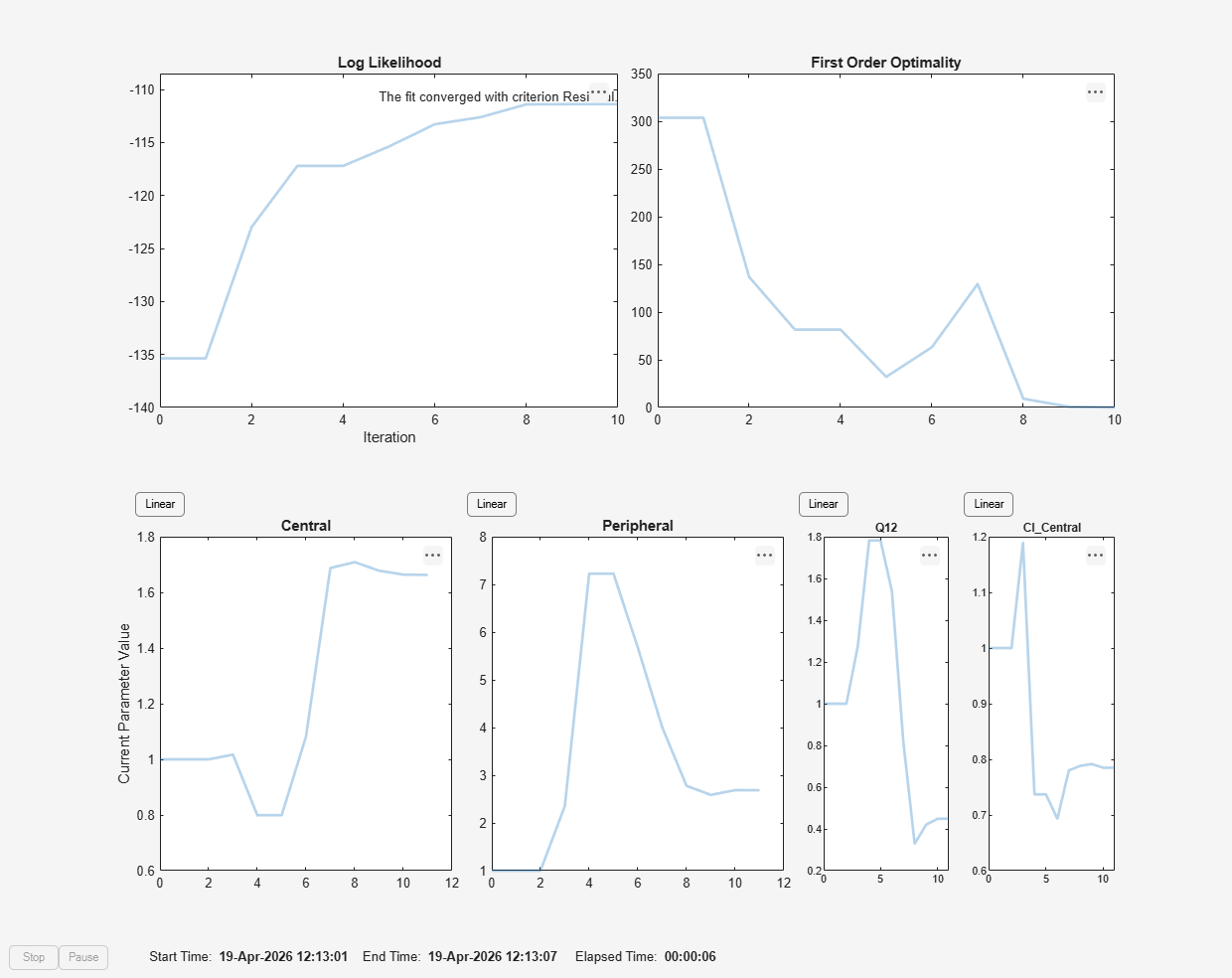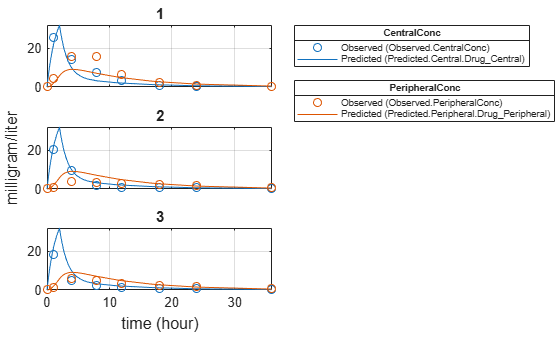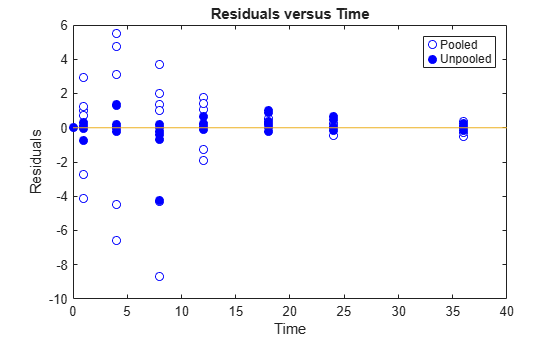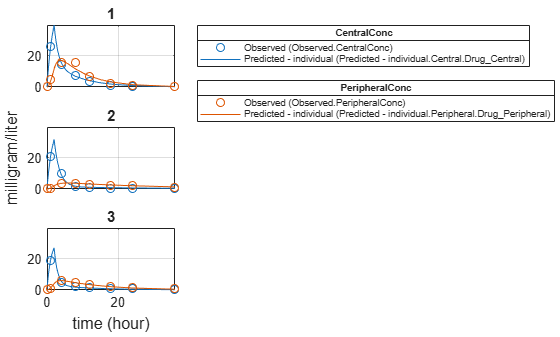# fit

Perform parameter estimation using SimBiology problem object

Since R2021b

## Syntax

``fitResults = fit(problemObject)``
``[fitResults,simdataI] = fit(problemObject)``
``[fitResults,simdataI,simdataP] = fit(problemObject)``

## Description

example

````fitResults = fit(problemObject)` performs parameter estimation using the model, data, and options defined by `problemObject` and returns the fitted results.```
````[fitResults,simdataI] = fit(problemObject)` also returns simulation data `simdataI` using the estimated parameter values. If `problemObject.FitFunction` is `"sbiofitmixed"`, simulations use the individual parameter estimates.```
````[fitResults,simdataI,simdataP] = fit(problemObject)` also returns simulation results using population parameter estimates. This syntax is supported only when `problemObject.FitFunction` is `"sbiofitmixed"`.```

## Examples

collapse all

This example shows how to estimate PK parameters of a SimBiology model using a problem-based approach.

Load a synthetic data set. It contains drug plasma concentration data measured in both central and peripheral compartments.

`load('data10_32R.mat')`

Convert the data set to a `groupedData` object.

```gData = groupedData(data); gData.Properties.VariableUnits = ["","hour","milligram/liter","milligram/liter"];```

Display the data.

```sbiotrellis(gData,"ID","Time",["CentralConc","PeripheralConc"],... Marker="+",LineStyle="none");```Use the built-in PK library to construct a two-compartment model with infusion dosing and first-order elimination. Use the configset object to turn on unit conversion.

```pkmd = PKModelDesign; pkc1 = addCompartment(pkmd,"Central"); pkc1.DosingType = "Infusion"; pkc1.EliminationType = "linear-clearance"; pkc1.HasResponseVariable = true; pkc2 = addCompartment(pkmd,"Peripheral"); model2cpt = construct(pkmd); configset = getconfigset(model2cpt); configset.CompileOptions.UnitConversion = true;```

Assume every individual receives an infusion dose at time = 0, with a total infusion amount of 100 mg at a rate of 50 mg/hour. For details on setting up different dosing strategies, see Doses in SimBiology Models.

```dose = sbiodose("dose","TargetName","Drug_Central"); dose.StartTime = 0; dose.Amount = 100; dose.Rate = 50; dose.AmountUnits = "milligram"; dose.TimeUnits = "hour"; dose.RateUnits = "milligram/hour";```

Create a problem object.

`problem = fitproblem`
```problem = fitproblem with properties: Required: Data: [0x0 groupedData] Estimated: [1x0 estimatedInfo] FitFunction: "sbiofit" Model: [0x0 SimBiology.Model] ResponseMap: [1x0 string] Optional: Doses: [0x0 SimBiology.Dose] FunctionName: "auto" Options: [] ProgressPlot: 0 UseParallel: 0 Variants: [0x0 SimBiology.Variant] sbiofit options: ErrorModel: "constant" Pooled: "auto" SensitivityAnalysis: "auto" Weights: [] ```

Define the required properties of the object.

```problem.Data = gData; problem.Estimated = estimatedInfo(["log(Central)","log(Peripheral)","Q12","Cl_Central"],InitialValue=[1 1 1 1]); problem.Model = model2cpt; problem.ResponseMap = ["Drug_Central = CentralConc","Drug_Peripheral = PeripheralConc"];```

Define the dose to be applied during fitting.

`problem.Doses = dose;`

Show the progress of the estimation.

`problem.ProgressPlot = true;`

Fit the model to all of the data pooled together: that is, estimate one set of parameters for all individuals by setting the `Pooled` property to `true`.

`problem.Pooled = true;`

Perform the estimation using the `fit` function of the object.

`pooledFit = fit(problem);`Display the estimated parameter values.

`pooledFit.ParameterEstimates`
```ans=4×3 table Name Estimate StandardError ______________ ________ _____________ {'Central' } 1.6627 0.16569 {'Peripheral'} 2.6864 1.0644 {'Q12' } 0.44945 0.19943 {'Cl_Central'} 0.78497 0.095621 ```

Plot the fitted results.

`plot(pooledFit);`Estimate one set of parameters for each individual and see if the parameter estimates improve.

```problem.Pooled = false; unpooledFit = fit(problem);```Display the estimated parameter values.

`unpooledFit.ParameterEstimates`
```ans=4×3 table Name Estimate StandardError ______________ ________ _____________ {'Central' } 1.422 0.12334 {'Peripheral'} 1.5619 0.36355 {'Q12' } 0.47163 0.15196 {'Cl_Central'} 0.5291 0.036978 ```
```ans=4×3 table Name Estimate StandardError ______________ ________ _____________ {'Central' } 1.8322 0.019672 {'Peripheral'} 5.3364 0.65327 {'Q12' } 0.2764 0.030799 {'Cl_Central'} 0.86035 0.026257 ```
```ans=4×3 table Name Estimate StandardError ______________ ________ _____________ {'Central' } 1.6657 0.038529 {'Peripheral'} 5.5632 0.37063 {'Q12' } 0.78361 0.058657 {'Cl_Central'} 1.0233 0.027311 ```
`plot(unpooledFit);`Generate a plot of the residuals over time to compare the pooled and unpooled fit results. The figure indicates unpooled fit residuals are smaller than those of the pooled fit, as expected. In addition to comparing residuals, other rigorous criteria can be used to compare the fitted results.

```t = [gData.Time;gData.Time]; res_pooled = vertcat(pooledFit.R); res_pooled = res_pooled(:); res_unpooled = vertcat(unpooledFit.R); res_unpooled = res_unpooled(:); figure; plot(t,res_pooled,"o",MarkerFaceColor="w",markerEdgeColor="b") hold on plot(t,res_unpooled,"o",MarkerFaceColor="b",markerEdgeColor="b") refl = refline(0,0); % A reference line representing a zero residual title("Residuals versus Time"); xlabel("Time"); ylabel("Residuals"); legend(["Pooled","Unpooled"]);```As illustrated, the unpooled fit accounts for variations due to the specific subjects in the study, and, in this case, the model fits better to the data. However, the pooled fit returns population-wide parameters. As an alternative, if you want to estimate population-wide parameters while considering individual variations, you can perform nonlinear mixed-effects (NLME) estimation by setting `problem.FitFunction` to `sbiofitmixed`.

`problem.FitFunction = "sbiofitmixed";`
`NLMEResults = fit(problem);`Display the estimated parameter values.

`NLMEResults.IndividualParameterEstimates`
```ans=12×3 table Group Name Estimate _____ ______________ ________ 1 {'Central' } 1.4623 1 {'Peripheral'} 1.5306 1 {'Q12' } 0.4587 1 {'Cl_Central'} 0.53208 2 {'Central' } 1.783 2 {'Peripheral'} 6.6623 2 {'Q12' } 0.3589 2 {'Cl_Central'} 0.8039 3 {'Central' } 1.7135 3 {'Peripheral'} 4.2844 3 {'Q12' } 0.54895 3 {'Cl_Central'} 1.0708 ```

Plot the fitted results.

`plot(NLMEResults);`Plot the conditional weighted residuals (CWRES) and individual weighted residuals (IWRES) of model predicted values.

`plotResiduals(NLMEResults,'predictions')`## Input Arguments

collapse all

SimBiology estimation problem, specified as a `fitproblem` object.

## Output Arguments

collapse all

Estimation results, returned as a scalar `OptimResults` object, `NLINResults` object, vector of `OptimResults` or `NLINResults` objects, or scalar `NLMEResults` object.

The returned results object type varies depending on if you used `problemObject.FitFunction="sbiofit"` or `problemObject.FitFunction="sbiofitmixed"`.

• If `FitFunction="sbiofit"` and `FunctionName="nlinfit"`, the returned results object type is `NLINResults`. For other optimization functions, the returned object type is `OptimResults` .

• If `FitFunction="sbiofitmixed"`, the returned object type is always `NLMEResults`.

When you use `FitFunction="sbiofit"`, the function returns either a scalar results object or vector of results objects as follows.

For an unpooled fit, the function fits each group separately using group-specific parameters, and `fitResults` is a vector of results objects with one results object for each group.

For a pooled fit, the function performs fitting for all individuals or groups simultaneously using the same parameter estimates, and `fitResults` is a scalar results object.

When the pooled option is not specified, and `CategoryVariableName` values of `estimatedInfo` objects are all `<none>`, `fitResults` is a single results object. This is the same behavior as a pooled fit.

When the pooled option is not specified, and `CategoryVariableName` values of `estimatedInfo` objects are all `<GroupVariableName>`, `fitResults` is a vector of results objects. This is the same behavior as an unpooled fit.

In all other cases, `fitResults` is a scalar object containing estimated parameter values for different groups or categories specified by `CategoryVariableName`.

See the Pooled property for details on how to perform a pooled, unpooled, or category fit.

When you use `FitFunction="sbiofitmixed"`, the function always returns a scalar `NLMEResults` object.

Simulation results, returned as a vector of `SimData` objects representing simulation results for each group (or individual) using individual-specific parameter estimates.

The states reported in `simDataI` are the states that are included in `problemObject.ResponseMap` as well as any other states listed in the `StatesToLog` property of the runtime options (`RuntimeOptions`) of the SimBiology model `problemObject.Model`.

Simulation results, returned as a vector of `SimData` objects representing simulation results for each group (or individual) using only fixed-effect estimates (population parameter estimates).

The states reported in `simDataP` are the states that are included in `problemObject.ResponseMap` as well as any other states listed in the `StatesToLog` property of the runtime options (`RuntimeOptions`) of the SimBiology model `problemObject.Model`.

## Version History

Introduced in R2021b